# Slow-Wave Structures

Click here to go to our page on group and phase velocity

Slow wave structures act to reduce the group velocity of a transmission line, or increase its group delay compared to a normal "fast-wave" structure. Slow-wave structures are useful in shrinking electrically-long elements. In microwave engineering, cost is a linear function of circuit area, so any tricks we can employ to shrink designs are worth knowing about. One of the applications of slow-wave circuits is in time delay networks.

Let's start the topic by providing the following definition:

Slowing factor: the ratio of the wave wavelength of a "native" transmission line to the wavelength in a slow-wave structure. The higher the slow-wave factor, the more the transmission line's wavelength is reduced compared to its "normal" wavelength in the chosen dielectric medium. By "normal" we are comparing it to CPW or microstrip or stripline at fixed impedance.

Note: in some references the slowing factor may be a number less than one, in that case it is the reciprocal of how we define it here.

### Cross-tie slow-wave circuits

This class of circuits are related to "artificial" transmission lines (an artificial transmission line uses lumped inductors and capacitors that obey the telegraphers equations). Note that there are many physical implementations of slow-wave structures that use this technique, all that is requires is a periodic structure of electrically short high-impedance and low-impedance lines.

The cross-tie slow-wave structure was first described by Seki and Hasegawa, in a paper titled Cross Tie Slow-Wave Coplanar Waveguide on Semi-Insulating GaAs Substrates published in Electronics Letters of the IEE (now IET), 10 December 1981, Volume 17 No 25 . It was further developed as a structure for IBM's silicon CMOS (or SiGe) circuits in the 2004 IEEE International Solid-State Circuits Conference paper titled On-Chip Interconnect for mm-Wave Applications Using an All-Copper Technology and Wavelength Reduction . Below are Figures 1 and 3 from the Seki and Hasegawa paper, to show you how the original structure was implemented.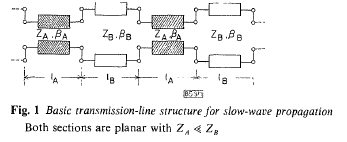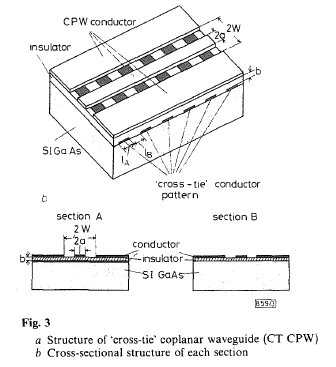The cross-tie slow-wave structure replaces a continuous transmission line with an artificial transmission line made up of many electrically-small segments. The segment impedance alternates from ZA to ZB along the chain. One impedance is lower than Z0, the other is higher than Z0. The high impedance section was created using a CPW structure with very wide gaps, while the low impedance section was created by the cross-tie capacitance shunting the center conductor to the dual grounds, which is actually a three capacitor network: one is underneath the conductor, and two are underneath the ground strips.

In order for this type of circuit to remain impedance matched, the impedances must obey the following relationship to Z0 according to Seki and Hazegawa: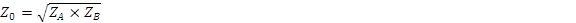According to this convention, it follows that: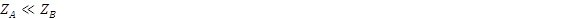The impedance matched condition is valid only if E1=E2 (the strip lengths are equal). Thus there are four degrees of freedom you can play with.

Seki and Hazegawa define K as the ratio of the two impedances: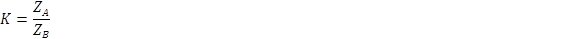Then they define the slow-wave factor as: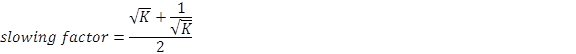If you want to torture yourself, try to solve for K....

As a convention, ZA<Z0<ZB so that K is a number greater than unity. The plot below shows the slow-wave factor as a function of ZB/ZA. Note that very high factors require extremely disparate line impedances; a slowing factor of 5 would require Z1=~5 ohms, Z2=~500 ohms (Z2/Z1=~100) for Z0=50 ohms. In practice, the maximum high impedance of a given media will be the limitation, you will never get 500 ohms, but you might get 125 ohms. Achieving almost any low impedance is easy, if you can work a thin-film capacitor onto your substrate.

You can refer to the cross ties as "microwave speed bumps" when you talk to your managers, so they can have at least a tiny understanding of what you are trying to do. Just don't flatter yourself that you understand how it works. If you are able to derive the slowing factor from Floquet's Theorem (like Seki and Hazagawa did) you should be a college professor, not an engineer.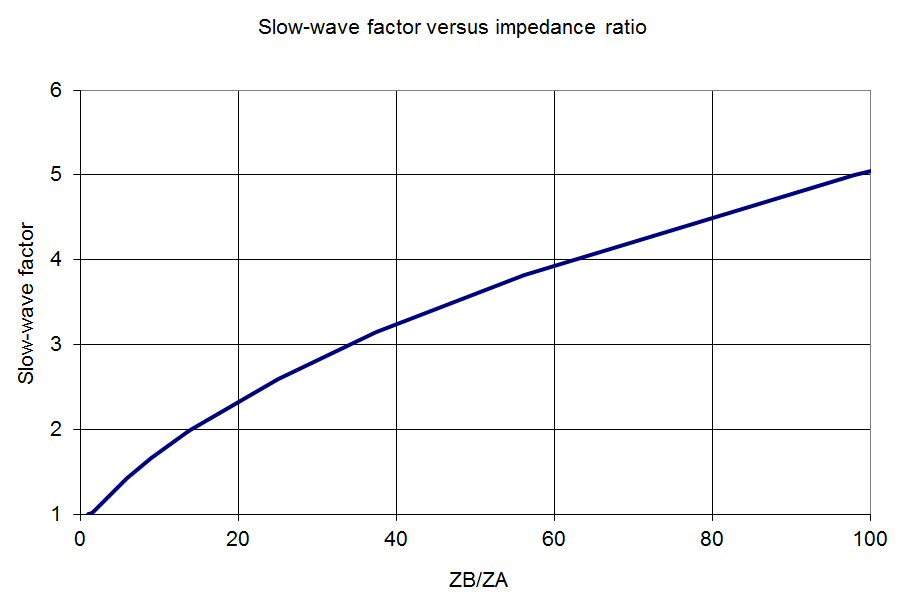The plot below separates out the two impedances versus slowing factor. Note the lines are scaled on opposite axes. A slowing factor of 2 in 50 ohm system requires K=13.92 (found by trial and error, not by solving that ugly equation), ZA=13.4, ZB=186.6 ohms.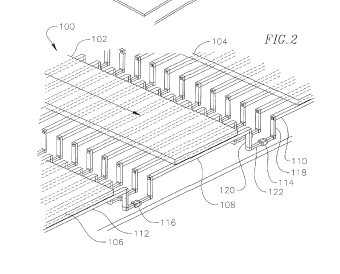Here's a figure of a unique slow-wave structure that was recently patented (US 8,264,300), inventor is Terry Cisco. Mr. Cisco was kind enough to reference this page on Microwaves101 in his patent, so we will pay him back by discussing his idea here. Terry's idea uses the BEOL metals on a CMOS or BiCMOS process to establish a CPW structure that has metallic "railroad ties" that it traverses. The individual cross ties are connected to NMOS transistors which can provide two states. When they are on, the ties add periodic capacitance to the line. When they are off, they will reduce the capacitance of the tie (increasing the ZA term. By switching in and out a certain number of ties, the wave can be slowed such that it provides a specific phase shift. Therefore this idea can be used as a digital phase shifter, with extremely small LSB (switching one tie could provide less than 1 degree phase shift for example). It should be noted that the characteristic impedance of the line will change with phase state. Also, the resistance of the NMOS FETs may change the loss of the line as a function of phase state.

US patent 7676903 is a MEMS version of Terry's idea, and the patent contains measure data which you might want to look at. Here you will see how the impedance match changes with phase state, as the line impedance is not maintained.

### Bragg frequency - what's that?

In the case of transmission lines, the onset of a stop band for electromagnetic waves in a periodic structure corresponds to Bragg reflection in crystals. In 1915, William Henry Bragg and William Lawrence Bragg developed the science of crystallography using X-ray diffraction. This father-and-son team is who we refer to when we talk of Bragg frequencies. Meet them in our Microwaves101 Hall of Fame!

In our example slow wave structure below, at the Bragg frequency, Z0 of the line becomes purely imaginary, propagation stops and the slow-wave structure ceases to function. The smaller the line segments, the higher the Bragg frequency. We have another Bragg frequency example, on an artificial transmission line, located here.

### Slow wave example

Below we have created a slow-wave transmission line in Microwave Office. It consists of eight high-impedance lines (186.6 ohms) interspersed with eight low impedance lines (13.4 ohms). Those impedance were calculated to provide a slowing factor of 2X in fifty ohm system. If you take the square root of ZA*ZB you will get 50 ohms. which meets the condition of impedance match. Each of the line segments is 10 electrical degrees at 10 GHz, and we added a reference line of 160 electrical degrees to measure the slow-wave structure against.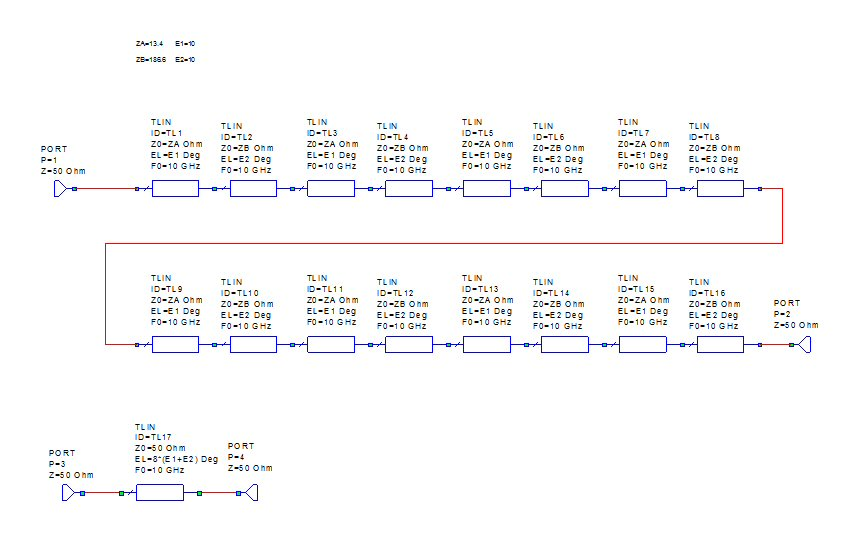In the S-parameters below, the Bragg frequency is shown to be approximately 30 GHz. At this frequency the individual ZA and ZB sections are 30 electrical degrees, or 1/12 wavelength. Adding or subtracting line sections does nothing to change the frequency, it is set by the lengths of the unit cell.

It is time for a Microwaves101 Rule of Thumb: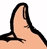In slow wave structures using alternate high and low impedance segments, the Bragg frequency occurs when the segments are 30 electrical degrees.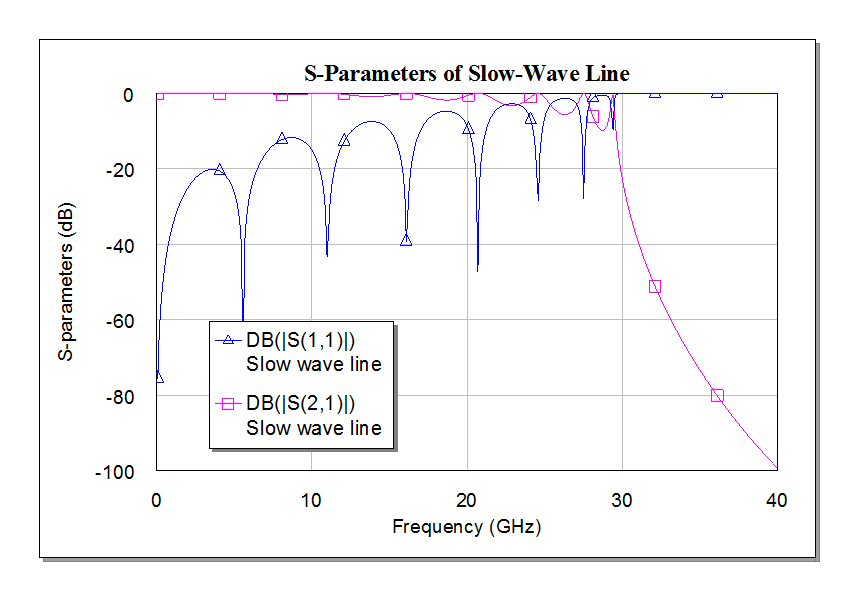The unwrapped phase also makes it obvious that the Bragg frequency of our example is near 30 GHz. Note that the slopes of the two lines differ by a factor of two, the slowing factor.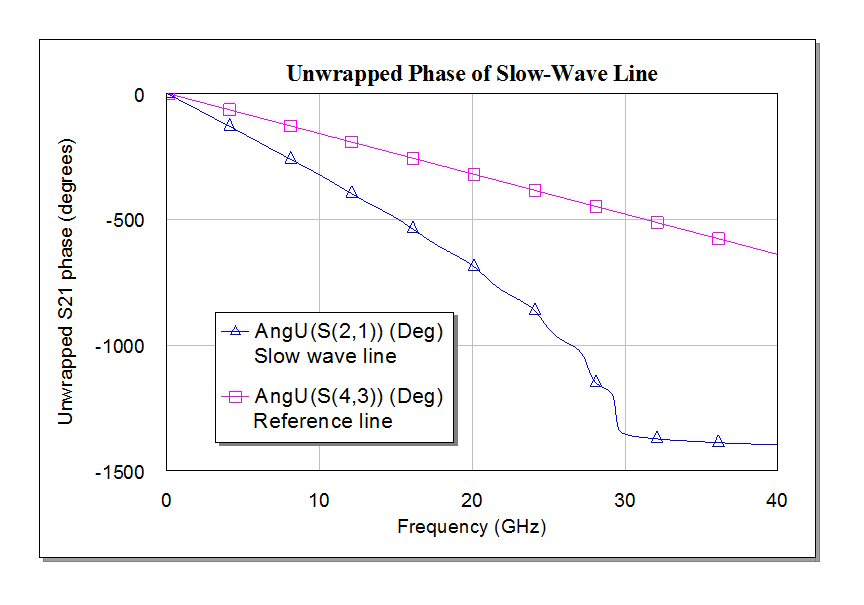Below the group delay of the slow wave versus the reference line are plotted. Yes, we have achieved a slowing factor of 2X, just like the math said we would.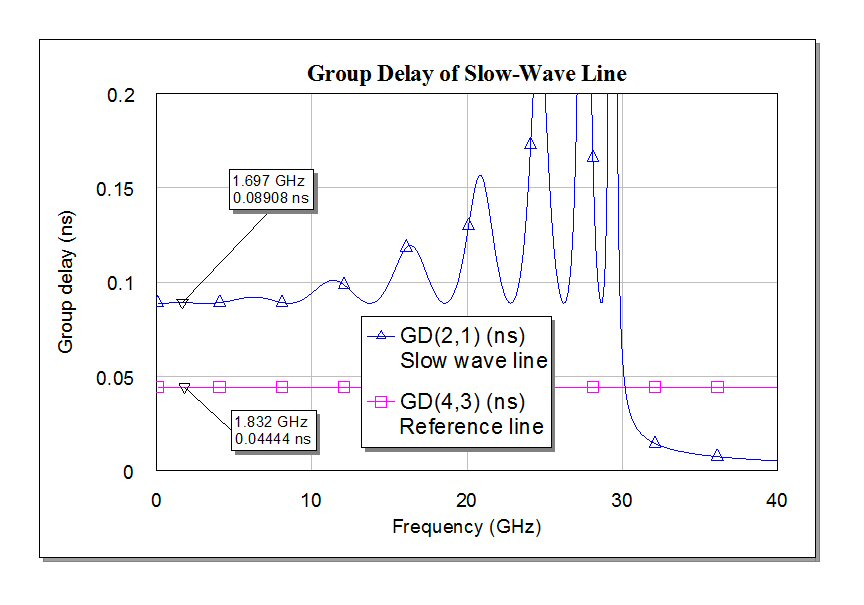Overall, the performance of the slow-wave structure example worked well up to about 5 GHz (pretty good impedance match, low ripple in delay). At this frequency the strips are just five electrical degrees. Thus we offer a second slow-wave rule of thumb:In slow wave structures using alternate high and low impedance segments, the line segments should be 5 electrical degrees or less at your maximum operating frequency.

One more thing... recall that we said that there are four degrees of freedom, as you can change the relative electrical lengths of the segments? In practice, the lengths of ZB (the high impedance line) will probably have to be longer than those of ZA. This is because the transition between impedances (the step) usually comes with parasitic capacitance. If you fix the two segments at the same length and build a circuit, don't blame us if the characteristic impedance is less than Z0....

### Metal-insulator-semiconductor structures (MIS)

This type of slow-wave structure is less well known.

Silicon is usually a very poor insulator. By creating a microstrip line on top of silicon, with a dielectric layer such as silicon dioxide in between, a strange thing can happen. The electric field does not penetrate into the silicon (which tries to shorts it out) but the magnetic field does. The separation of electrical and magnetic energy slows the wave propagation. Substantial reduction in wavelength is possible. Here's the earliest reference to the topic that we could find:

IEEE Hasegawa and Furukawa, Slow-wave propagation along a microstrip line on Si-SiO2 system, IEEE Proceedings, 1979, pp 297-299.

GaAs can also be used to create a MIS slow-wave structure. In this case an epitaxial layer is grown on top of the wafer, because GaAs is such a good semi-insulating substrate. A layer of low-loss dielectric is added on top (silicon dioxide, silicon nitride, etc.) Here's the original reference on the subject:

Hasegawa and Okizaki, M.I.S. and Schottky Slow Wave Coplanar Striplines on GaAs Substrates, IEE Electronics Letters, 27th October 1977, Vol.13, No 20.

Author : Unknown Editor